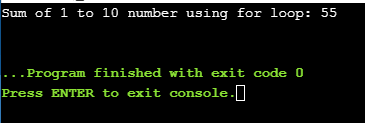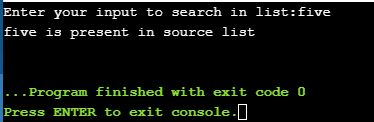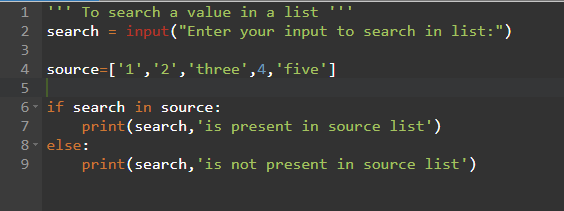## Pages

### For loop - Python practice program

For loop - Python practice program:

Copy and try on your own.
1) Display sum 1 to 10 number by using for loop

code:
'''Display sum 1 to 10 number by using for loop'''
n=10
ans=0
for i in range(1,n+1):
ans+=i
print("Sum of 1 to 10 number using for loop:",ans)Display sum 1 to 10 number by using for loop
Output:Output of display sum 1 to 10 number
2) Program to search a value in a list

We can code this logic using simple if condition also.

code:
''' To search a value in a list using for loop'''
search = input("Enter your input to search in list:")

source=['1','2','three','4','five']
count=0
for i in source:
if i == search:
print(search,'is present in source list')
count+=1
if count == 0:
print(search,'is not present in source list')Search value in a list using for loop
Output:Output of search value in a list
Method 2: using simple if-elseSearch value in a list using if-else